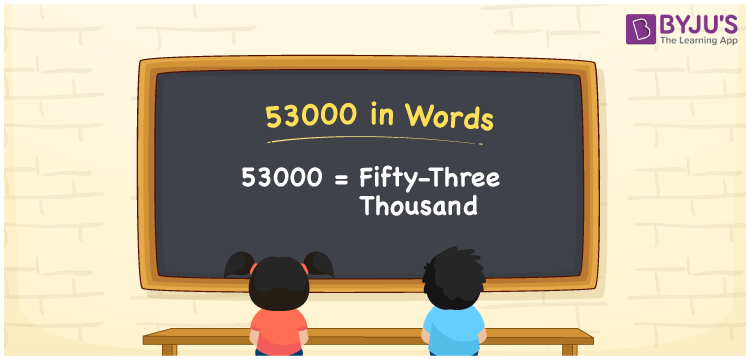# 53000 in Words

The number name of 53000 is fifty-three thousand. For converting numbers to words, the place value chart is important. As a result, we may conclude that using the place value table when writing 53000 in words is beneficial. For example, if you received Rs. 53000 in six months, you may write “I received Rs. 53000 in six months”. Let us look at how to write 53000 in words and the steps needed with a complete explanation.

 53000 in Words: Fifty-three Thousand. Fifty-three Thousand in Numerical Form: 53000.

## 53000 in English Words## How to Write 53000 in Words?

To convert 53000 to words, we can utilize a place value chart. Below is a place value table for the number 53000, as well as an expanded version.

 Ten-thousands Thousands Hundreds Tens Ones 5 3 0 0 0

Using place value chart, the number 53000 is represented as follows:

= 5 × Ten thousand + 3 × Thousand + 0 × Hundred + 0 × Ten + 0 × One

= 5 × 10000 + 3 × 1000

= 50000 + 3000

= 53000

= fifty-three thousand

Hence, 53000 in words is fifty-three thousand.

The natural number 53000 comes after 52999 but before 53001.

53000 in words – Fifty-three thousand

Is 53000 an odd number? – No

Is 53000 an even number? – Yes

Is 53000 a perfect square number? – No

Is 53000 a perfect cube number? – No

Is 53000 a prime number? – No

Is 53000 a composite number? – Yes

## Frequently Asked Questions on 53000 in Words

Q1

### Write 53000 in words.

53000 in words is fifty-three thousand.

Q2

### Simplify 54000 – 1000, and express in words..

Simplifying 54000 – 1000, we get 53000. Hence, 53000 in words is fifty-three thousand.

Q3

### Convert fifty-three thousand into numbers.

Fifty-three thousand in numbers is 53000.